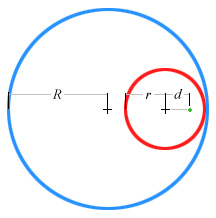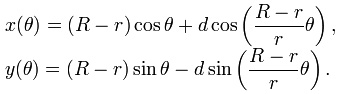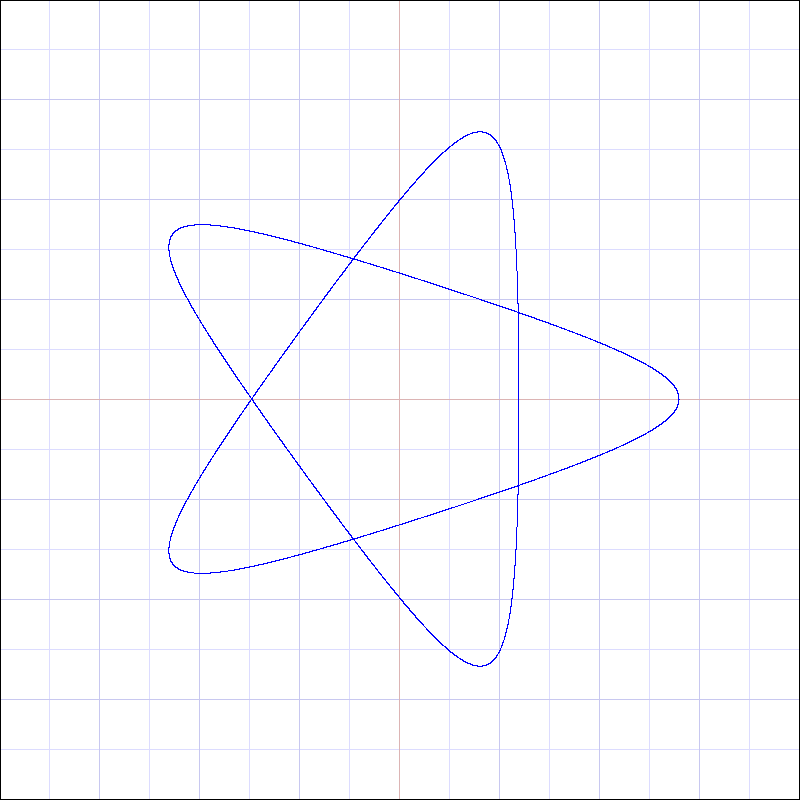R r d rotations
 New Graph     50 pixels per square

R = Radius of Outer Circle
r = Radius of Disk
d = Distance of point from the center of disk
rotations = number of rotations of the disk

(18849 Points plotted)